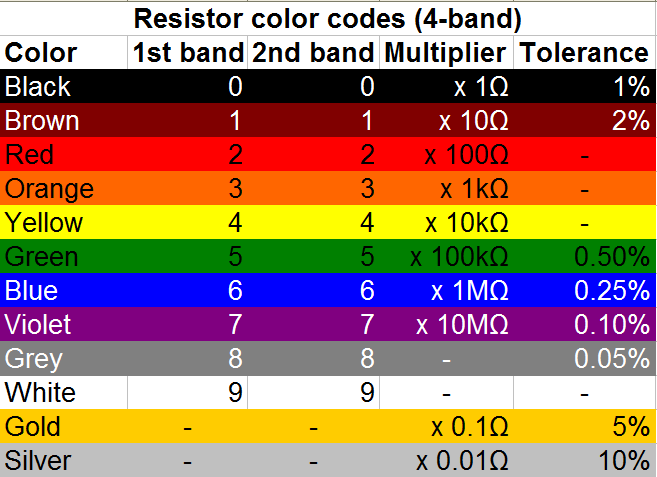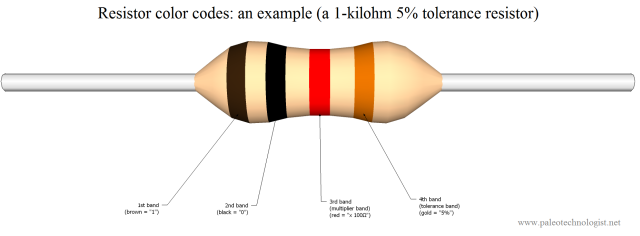# Components: Resistors

One of the most basic electronic components is the resistor. At first, these components don’t seem very useful; their function is to restrict (or resist) the flow of electrical current through a circuit. With today’s emphasis on efficiency, this seems pointless. However, various effects of resistors’ behavior make them important electronic components — and one of the easiest ones to understand. If you plan on building electronic circuits of more than the absolute minimum complexity, you will eventually need to understand and use resistors. Fortunately, this is easy.

Understanding resistors starts with an understanding of Ohm’s Law. (Voltage is equal to Current times Resistance. Follow the link above for a more in-depth explanation.)

Resistors come in many forms; perhaps the type most commonly used by hobbyists is the 1/4-watt, through-hole fixed-value resistor. These are axial components (meaning they have two connecting wires, initially 180 degrees apart), and are about 2mm in diameter at their thickest part (they would fit inside a typical drinking straw if not bent.) The example resistor shown below is of this type.

Typically, fixed-value through-hole resistors will have four color bands, which specify what the resistor’s nominal value and tolerance (margin of error) is. Here is a chart to help decode what the colors mean:A well-known mnemonic (which I did not write) is: "Bad Boys Ravish Our Young Girls, But Violet Gives Willingly."

Here is an example of how these codes would be read on a garden-variety 1-kilohm (or “1k”) resistor. (Click on the image below for a higher-resolution version.) Reading the bands from the left, the brown band represents 1, and the black band represents 0. Putting these together gives “10” for the base value. (The first band is almost never black, except in the unusual case of a “zero-ohm” resistor: a single black band, otherwise known as a “wire.”) The third band is the multiplier band; red signifies multiplication by 100, making the total value 10 x 100 = 1,000 ohms — or “1k.” The 4th band is the tolerance band; gold signifies that the true resistance value of this component should be within 5% of the stated value (950 to 1,050 ohms).A 1-kilohm resistor (10 + 0, times 100, is 1000 ohms). The wire is roughly as thick as a pencil lead. Click for larger.

Resistors are used for many tasks in electronics. Without going into too much detail, here is a sampling of some of their many uses:

• Current-limiting: a 470-ohm or similar resistor is typically needed in series with an LED, in order to limit the amount of current flowing though the LED.
• Voltage-divider use: Two or more resistors can be used to “divide” a voltage proportionately to the total resistance. For example, if a 2k and 3k resistor were placed in series between a 5V supply and ground, the voltage between the two would be 3V (if the 3k resistor was connected to ground) or 2V (if the 2k resistor was connected to ground).
• As a “pull-up” or “pull-down” resistor: The voltage on an unconnected (or “floating”) wire is affected by nearby electrical fields, and tends to pick up a lot of noise. If such a wire is connected to the input of a digital circuit, the results will be unpredictable. A fairly high value resistor (1k is typical) is used to “pull up” the voltage to a logical high voltage (typically 5V or 3.3V) or “pull down” the voltage to ground. A switch can then safely be used to connect the input to the other value, without shorting anything out (the current through the resistor is small enough to be negligible.)
• To measure current: A small, precise resistor (or strip of wire of known resistance) can be used to measure current passing through it. Since Voltage = Current times Resistance (V=IR), a current passing through a known resistance will produce a voltage differential directly proportional to that current. Passing, say, 3 amps through a 0.01-ohm shunt will produce a voltage of 30mV across the shunt. This can be measured by a voltmeter to infer the amount of current flowing through the shunt.
• To discharge a capacitor: Some capacitors can hold a large amount of energy for a long time. When high-power equipment is turned off and the supply cord disconnected, it is safest if any capacitors can be automatically discharged. A “large” resistor (meaning one with a large resistance value, such as several kilohms or megohms) is permanently wired in parallel with the capacitor. When the power is turned off, this “bleeder” resistor safely discharges the capacitor.
This entry was posted in Analog, Components, EET201, EET205, Electronics, Science and tagged , , , , . Bookmark the permalink.

### One Response to Components: Resistors

1.Peter Enmore says:

Knowing the color coding of resistors will make it much easier for one to understand the value of it.